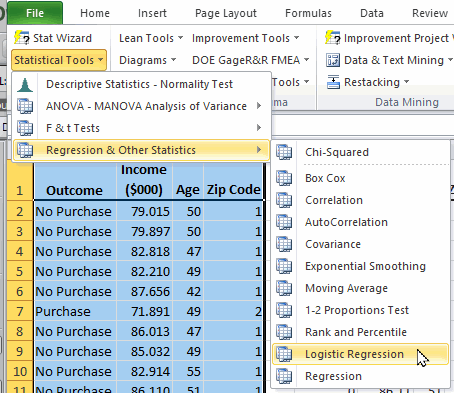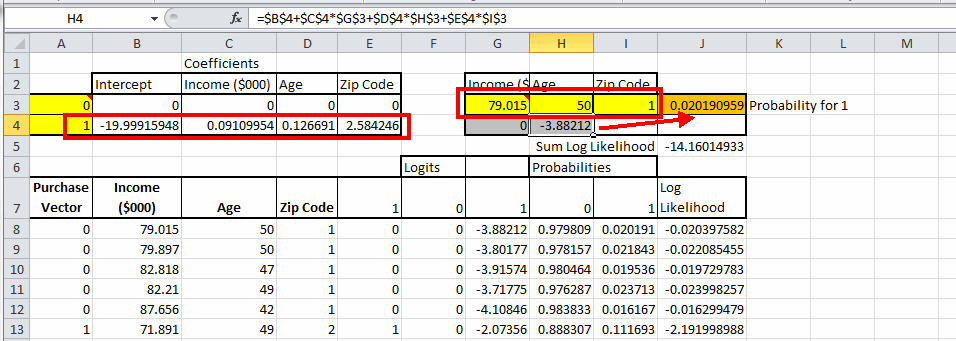# Need Binary Logistic Regression in Excel?

## QI Macros can do it for you!

#### Run Binomial Logistic Regression using QI Macros

2. Click on the QI Macros menu > Statistical Tools > Regression & Other Statistics > Logistic Regression.
3. QI Macros will do the math and analysis for you.

### What is Binary Logistic Regression Analysis?

Binary Logistic Regression estimates the probability of an event occurring (e.g., voted or didn't vote/passed or didn't pass), based on a given dataset of independent variables. Since the outcome is a probability, the dependent variable is bounded between 0 and 1.

### Example of Binary Logistic Regression in Excel using QI Macros

1. Select two or more columns of data (NOTE: The first column of your data must be setup as 1 (pass) or 0 (fail), purchase/no purchase, good/bad, etc. while the next column(s) in your data set should include your predictors):
2.The sample data shown above is found in QI Macros Help > Open QI Macros Sample Data > statistical.xlsx > "Binomial Logistic Regression" tab

3. Next, click on the QI Macros menu and choose Statistical Tools > Regression & Other Statistics > Logistic Regression (Binary):
4.Excel's Solver add-in is used to perform the Coefficients calculations.
If Solver is not enabled in your Excel, OR you are using a Mac, you will be provided with information about how to calculate the Coefficients manually, using Solver.

5. Evaluate the Binary Logistic Regression results:If we change the Zip Code to 2, the probability of a sale increases 10X:In this example:

• The probability of someone making a purchase is derived from past data.
• The income, age, and Zip Code can then be used to predict the probability that a person will purchase the product.

NOTE: We will offer both Binary Logistic Regression and Multinomial Logistic Regression in the October 2023 version of QI Macros.

*Logistic Regression functionality introduced in the July 2022 version of QI Macros*